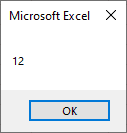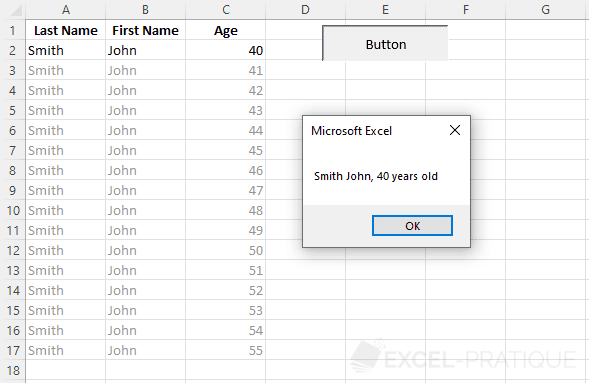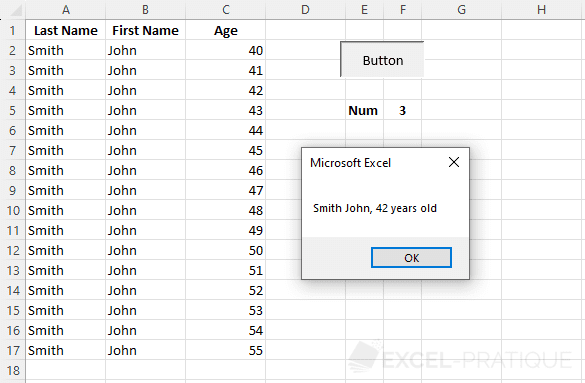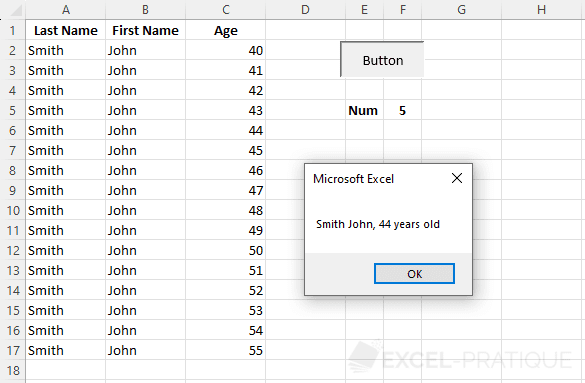VBA Course: Variables

Variables make it possible to store all sorts of information.

Here's the first example:

'Display the value of the variable in a dialog box
Sub variables()
'Declaring the variable
Dim my_variable As Integer
'Assigning a value to the variable
my_variable = 12
'Displaying the value of my_variable in a MsgBox
MsgBox my_variable
End Sub

This first line of code declares the variable (this is generally placed at the beginning of the procedure).

Dim my_variable As Integer
• Dim: declares the variable
• my_variable: the name chosen for this variable (no spaces allowed)
• As: declares the variable's type
• Integer: variable type

Declaring these variables is not absolutely necessary, but it is recommended. It makes it easier to find them, can help resolve problems, etc. In short, it's a good idea to get in the habit of declaring variables correctly.

A variable's type indicates the nature of its contents (text, numbers, date, etc.).

And then a value is given to the variable:

my_variable = 12

Finally, the value of the variable is displayed in a dialog box:

MsgBox my_variable

MsgBox "value" is the simplest way to display a value in a dialog box.

We'll go into more detail about dialog boxes in later lessons ...

The code will have this result:If you don't understand what the point of using these variables is yet, don't worry, the examples introduced in the following lessons will prove their usefulness ...

The types of variables

NameTypeDetailsSymbol
ByteNumericalWhole number between 0 and 255.
IntegerNumericalWhole number between -32'768 and 32'767.%
LongNumericalWhole number between - 2'147'483'648 and 2'147'483'647. &
CurrencyNumericalFixed decimal number between -922'337'203'685'477.5808 and 922'337'203'685'477.5807.@
SingleNumericalFloating decimal number between -3.402823E38 and 3.402823E38.!
DoubleNumericalFloating decimal number between -1.79769313486232D308 and 1.79769313486232D308.#
StringTextText.\$
DateDateDate and time.
BooleanBooleanTrue or False.
ObjectObjectMicrosoft Object.
VariantAny typeAny kind of data (default type if the variable is not declared).

Some examples with different types of variables:

'Example: whole number
Dim nbInteger As Integer
nbInteger = 12345

'Example: decimal number
Dim nbComma As Single
nbComma = 123.45

'Example: text
Dim varText As String
varText = "Excel-Pratique.com"

'Example: date
Dim varDate As Date
varDate = "06.04.2012"

'Example: True/False
Dim varBoolean As Boolean
varBoolean = True

'Example: object (Worksheet object for this example)
Dim varSheet As Worksheet
Set varSheet = Sheets("Sheet2") 'Set => assigning a value to an object variable

'Example of how to use object variables: activating the sheet
varSheet.Activate

The symbols in the table above can be used to shorten our variable declarations.

For reasons of readability, we will not be using these in the lessons, but here is an example anyway:

Dim example As Integer
Dim example%

These two lines are identical.

It's possible to force the declaration of variables by putting Option Explicit right at the beginning of a module (this way an error message will be displayed if you have forgotten to declare variables).

Practice exercise

We will now create, step by step, a macro that retrieves a last name from cell A2, a first name from cell B2, an age from cell C2, and displays them in a dialog box.

Source file: variable_exercise.xls

We'll begin by declaring the variables (all on the same line, separated by commas):

Sub variables()
'Declaring variables
Dim last_name As String, first_name As String, age As Integer

End Sub

Then we assign values to the variables using Cells:

Sub variables()
'Declaring variables
Dim last_name As String, first_name As String, age As Integer

'Variable values
last_name = Cells(2, 1)
first_name = Cells(2, 2)
age = Cells(2, 3)

End Sub

Finally, we'll display the results in a dialog box, using the & operator to join the values (as in Excel).

Sub variables()
'Declaring variables
Dim last_name As String, first_name As String, age As Integer

'Variable values
last_name = Cells(2, 1)
first_name = Cells(2, 2)
age = Cells(2, 3)

'Dialog box
MsgBox last_name & " " & first_name & ", " & age & " years old"
End Sub

The results:The next step is to display in a dialog box the row from the table that is indicated by the number in cell F5.

This is the goal:Take a moment to try to solve this problem yourself before looking at the solution below ...

.
.
.
.
.
.
.
.
.
.
.
.
.
.
.
.
.
.
.
.
.
.
.
.
.
.
.
.
.
.

The solution:

Sub variables()
'Declaring variables
Dim last_name As String, first_name As String, age As Integer, row_number As Integer

'Variable values
row_number = Range("F5") + 1
last_name = Cells(row_number, 1)
first_name = Cells(row_number, 2)
age = Cells(row_number, 3)

'Dialog box
MsgBox last_name & " " & first_name & ", " & age & " years old"
End Sub

'Declaring variables
Dim last_name As String, first_name As String, age As Integer, row_number As Integer

The variable row_number will now take the value of cell F5, to which we have added 1 (so that we don't get data from the first row, which contains the table's titles), so that row_number will have the row number of the cells that we are interested in as its value:

row_number = Range("F5") + 1

All that's left to do is to replace the row number in the Cells command with our variable:

last_name = Cells(row_number, 1)
first_name = Cells(row_number, 2)
age = Cells(row_number, 3)

Now our macro displays the row that we're interested in from the table.By the way, please note that we can reduce this procedure to a single line of code:

Sub variables()
MsgBox Cells(Range("F5")+1,1) & " " & Cells(Range("F5")+1,2) & ", " & Cells(Range("F5")+1,3) & " years old"
End Sub

Although the code will work perfectly, it is much less readable than the previous version and more difficult to rework (to make sure that our code is easy to understand, we won't be abbreviating it in this way in these lessons).